台北 花園大酒店 Taipei Garden Hotel

4,200

• 捷運西門站
• 西門町商圈

選擇專案房型及間數

更改 台北 花園大酒店 的空房情形及價格

•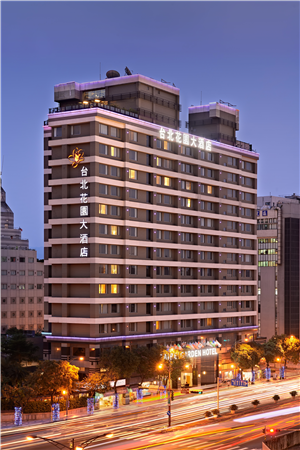酒店外觀
•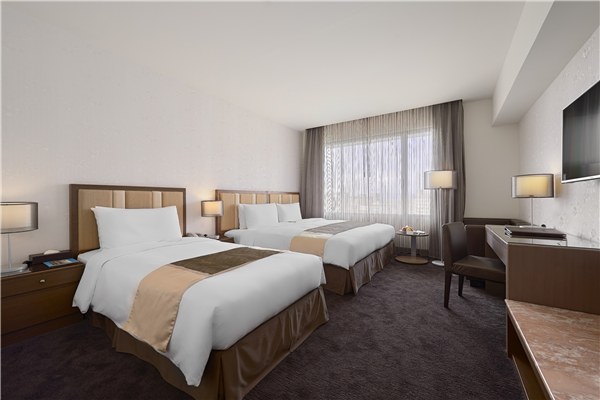客房
•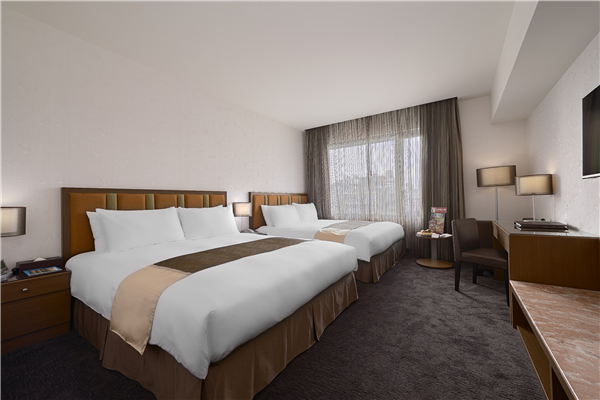客房
•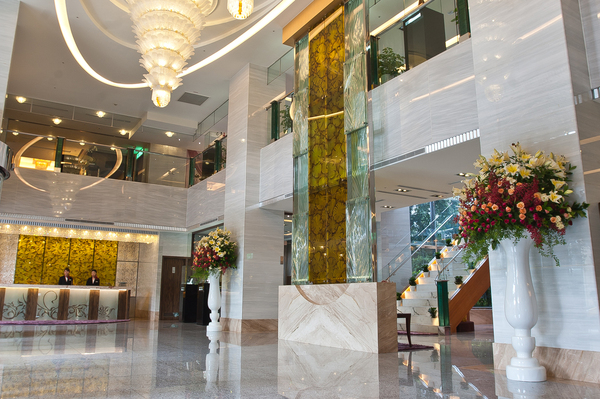環境
•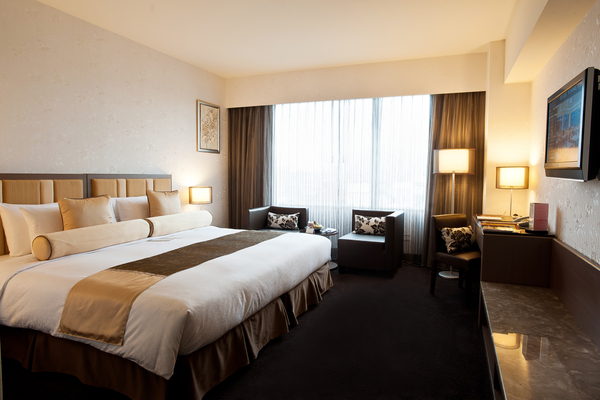客房
•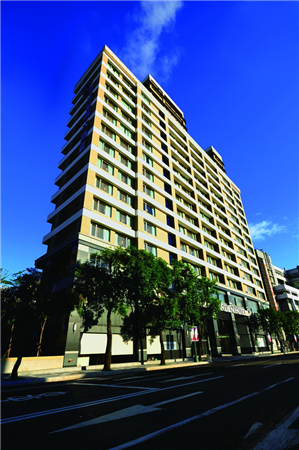酒店外觀
•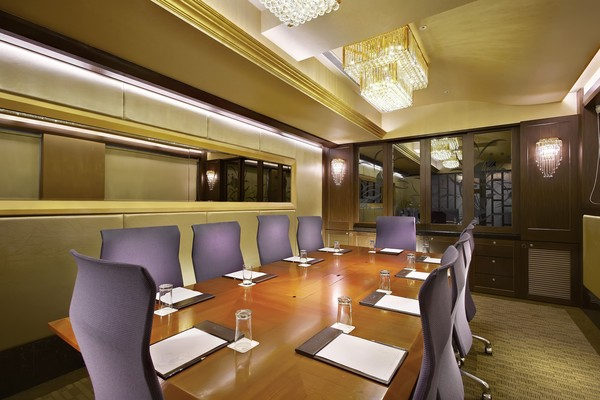環境
•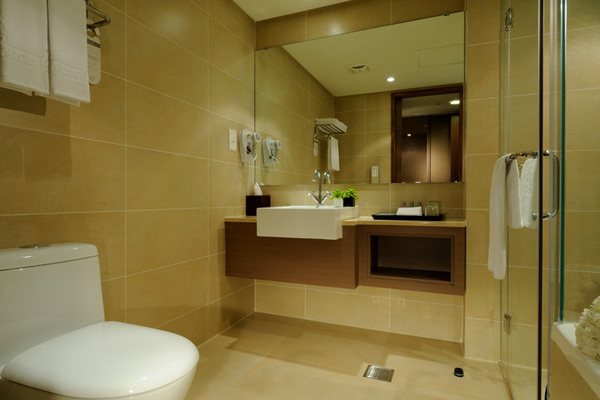客房
•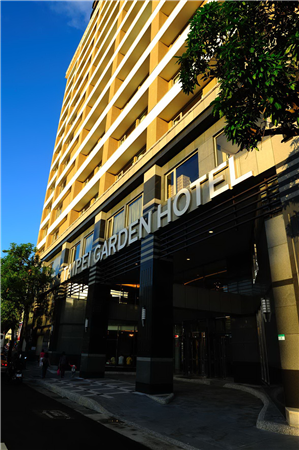入口
•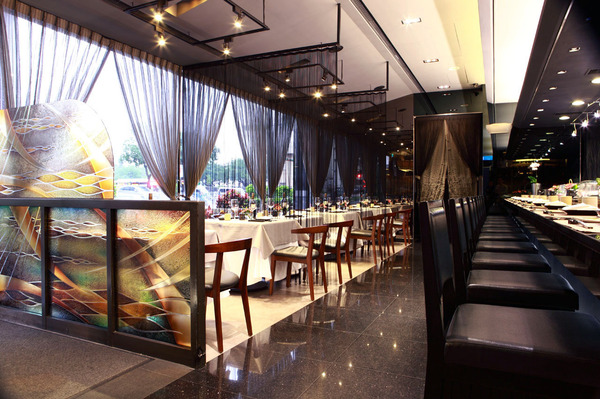環境
•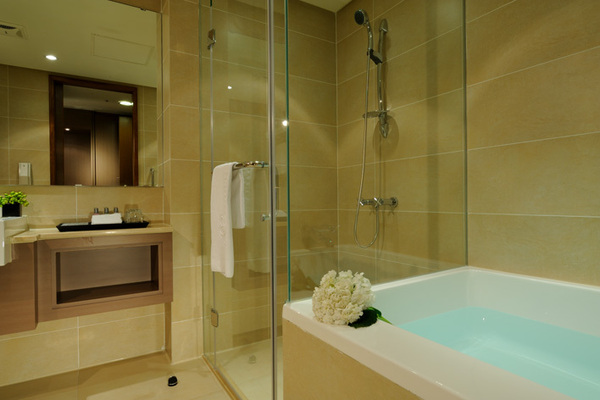客房
•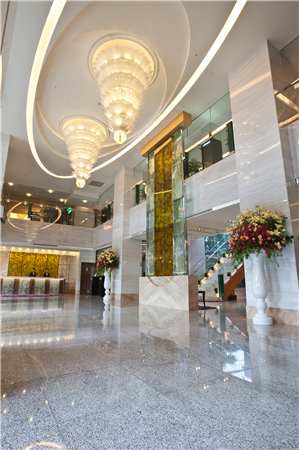大廳
•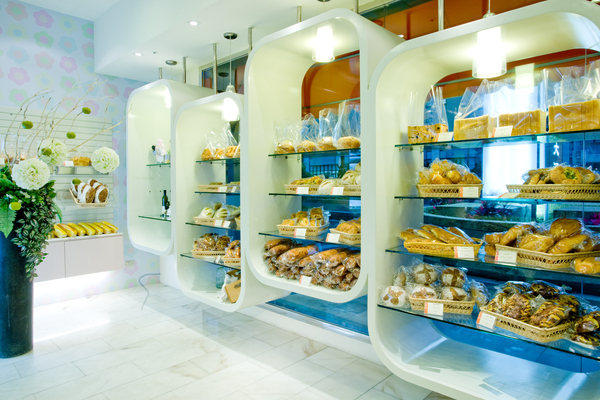環境
•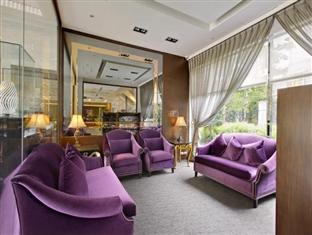商務中心
•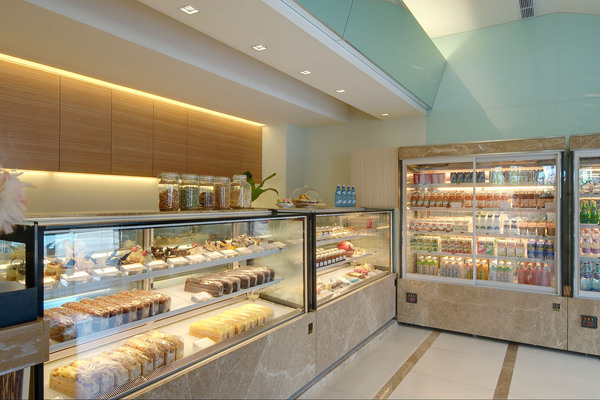環境
•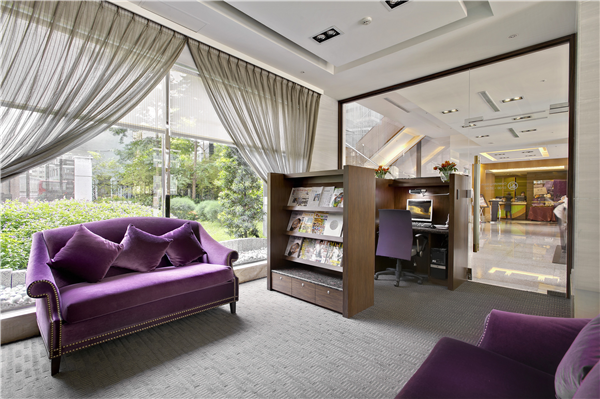商務中心
•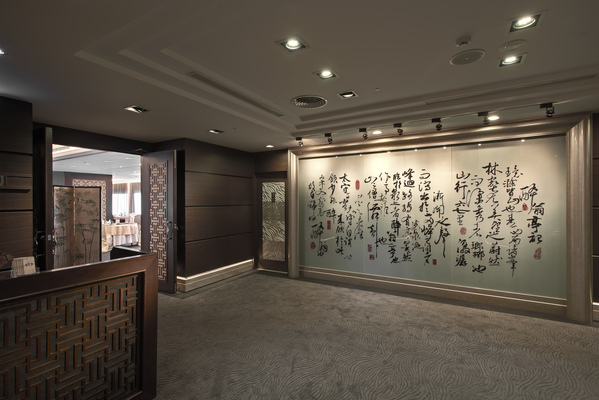環境
•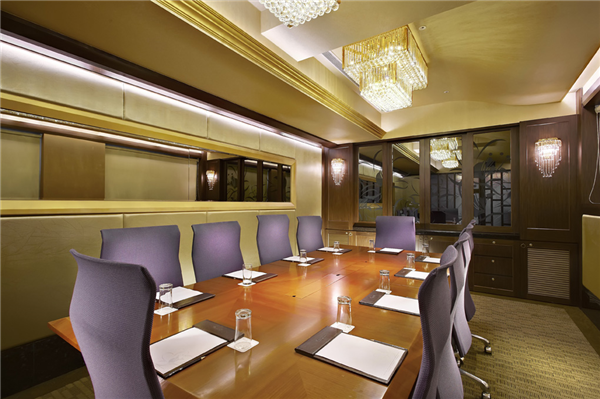會議室
•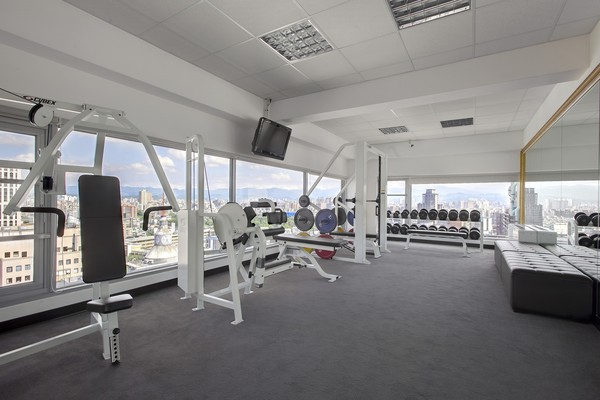環境
•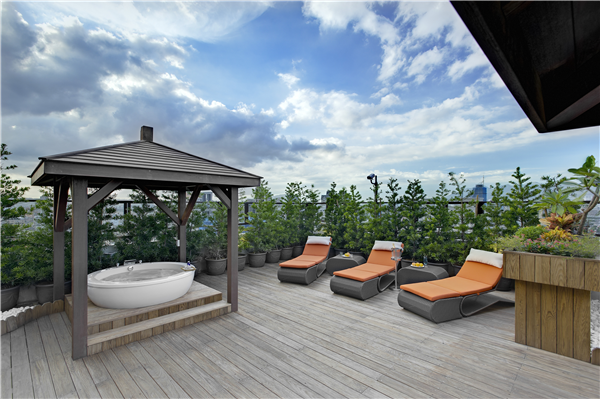漩渦按摩浴池
•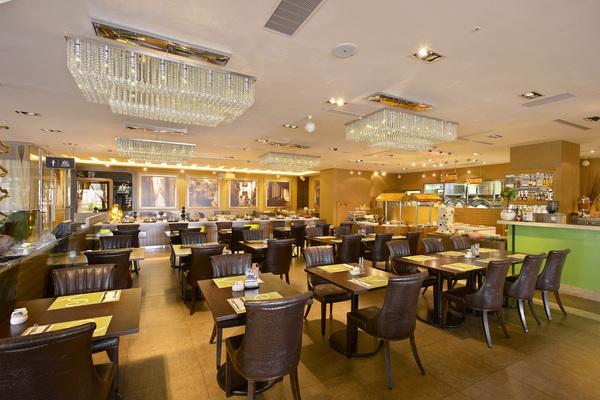環境
•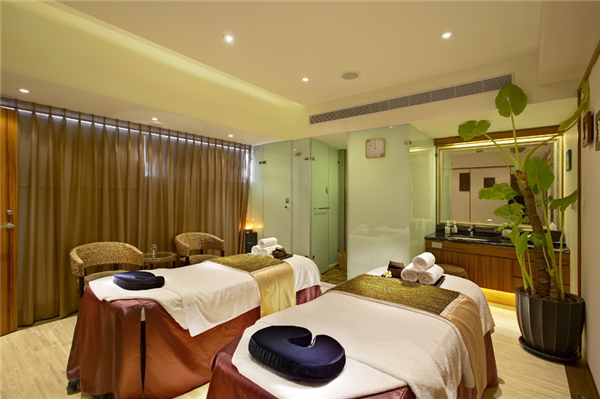水療中心
•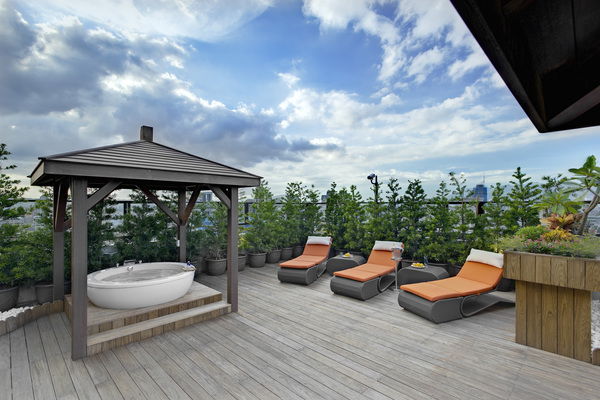環境
•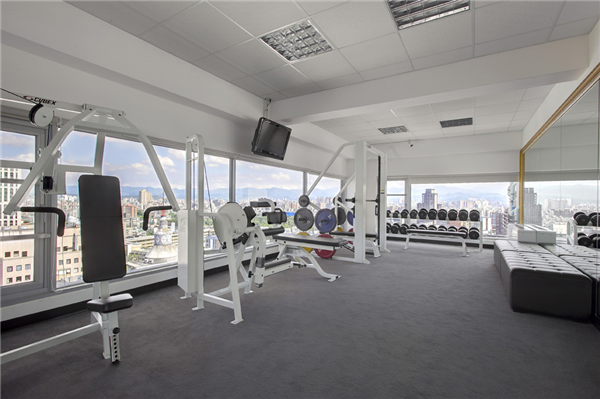健身房
•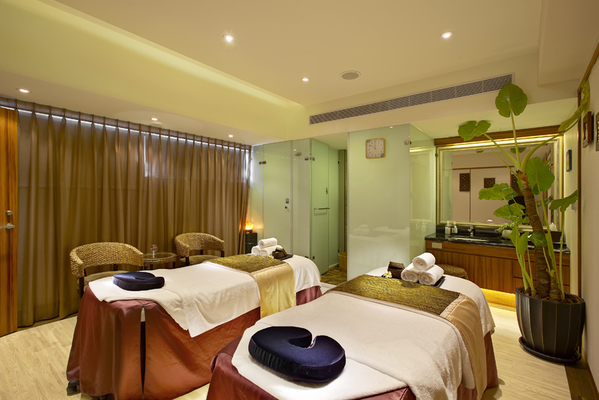環境
•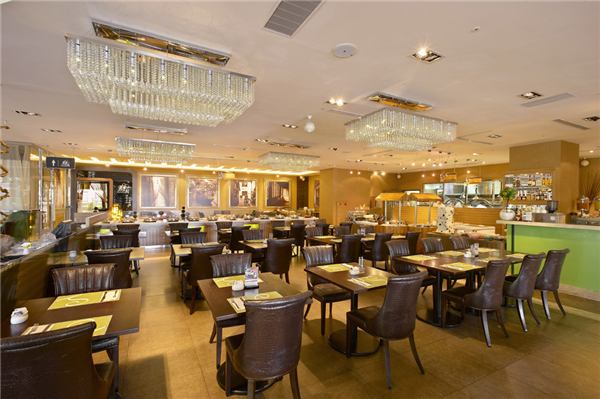餐廳
•環境
•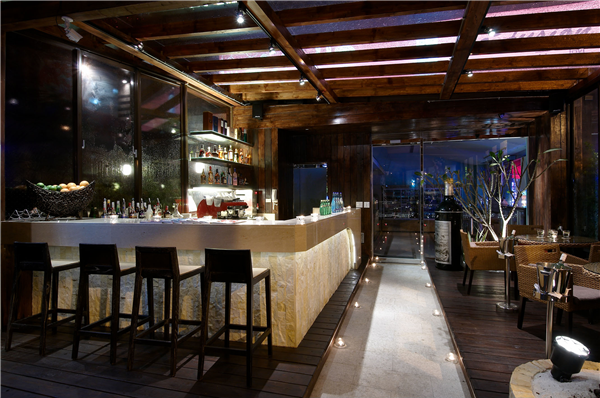酒吧/高級酒吧
•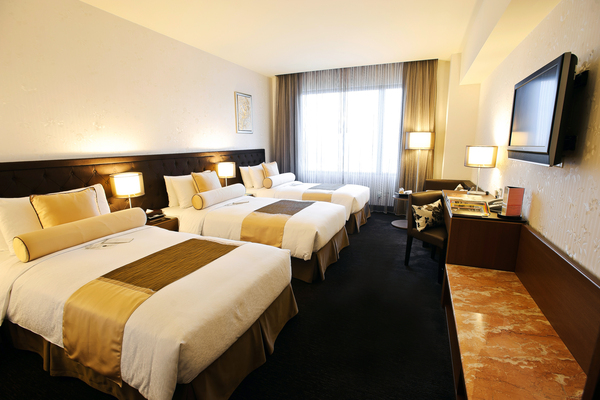環境
•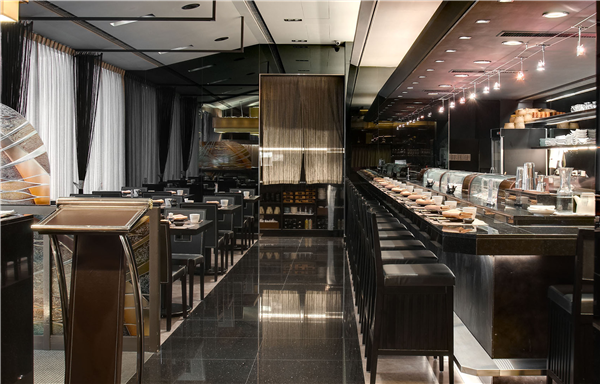日本餐廳
•環境
•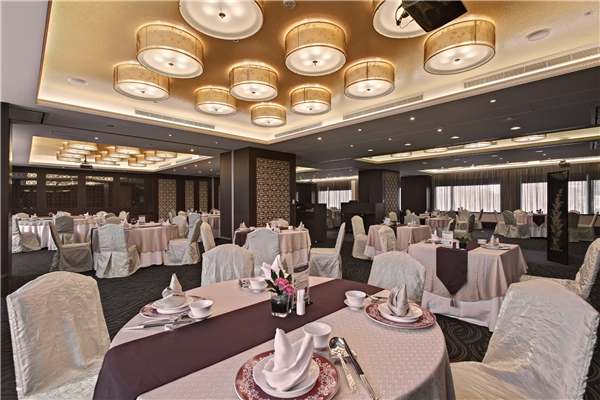中餐廳
•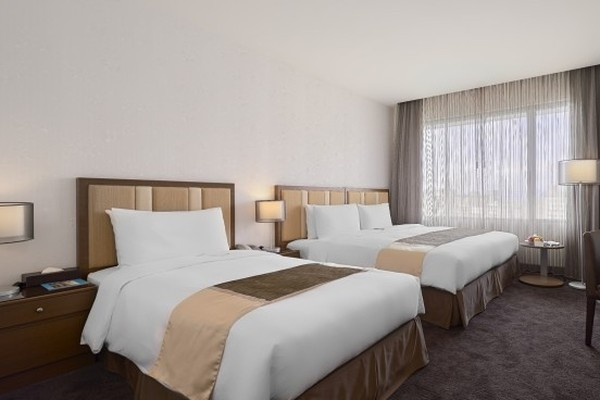環境
•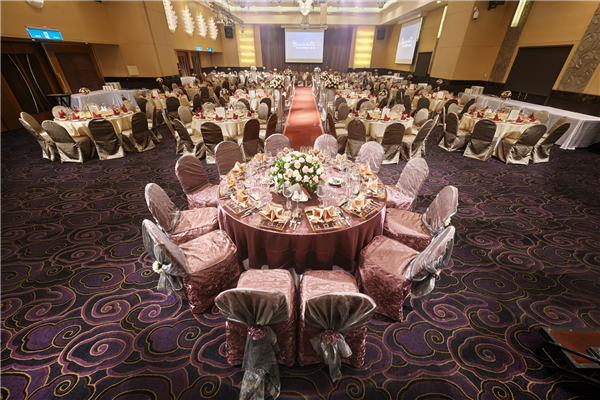宴會廳
•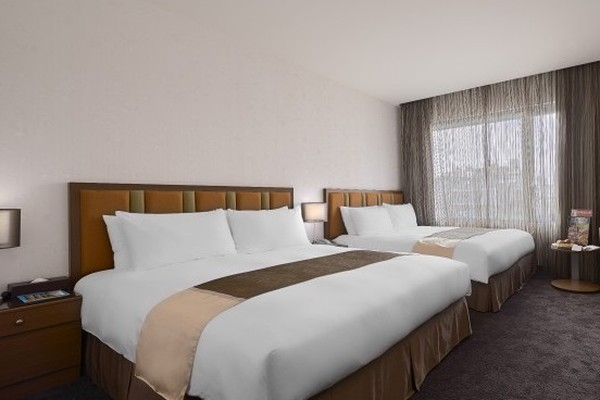環境
•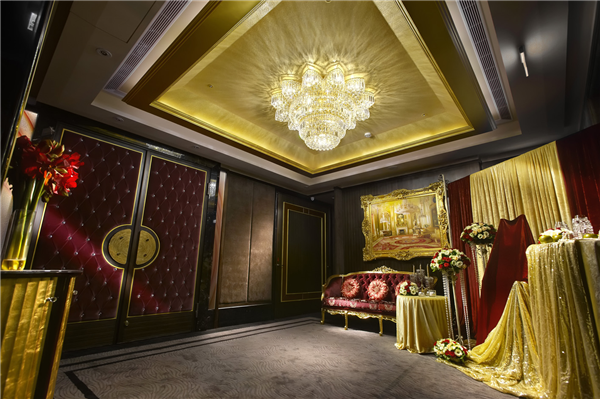宴會廳
•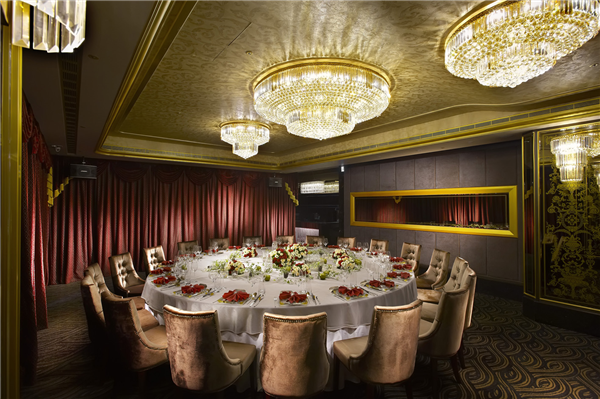宴會廳
•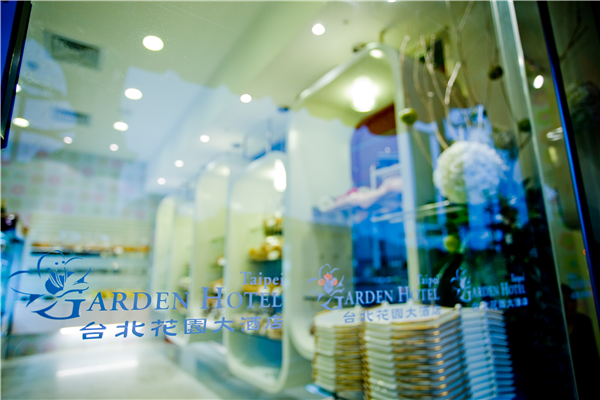商店
•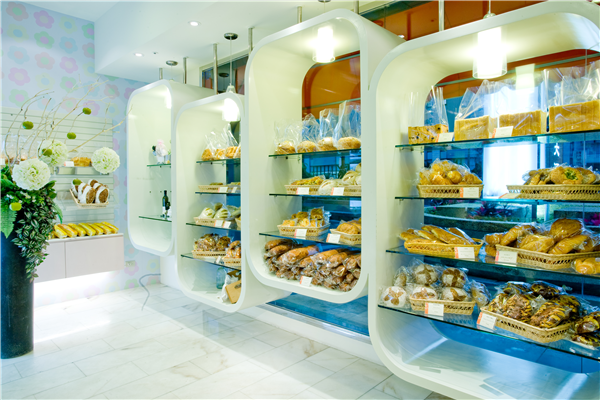商店
•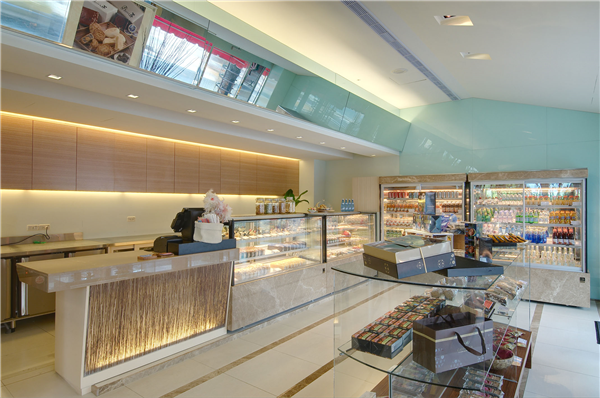商店
•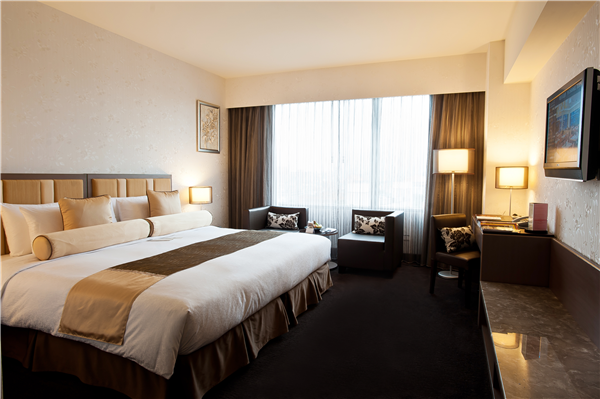客房
•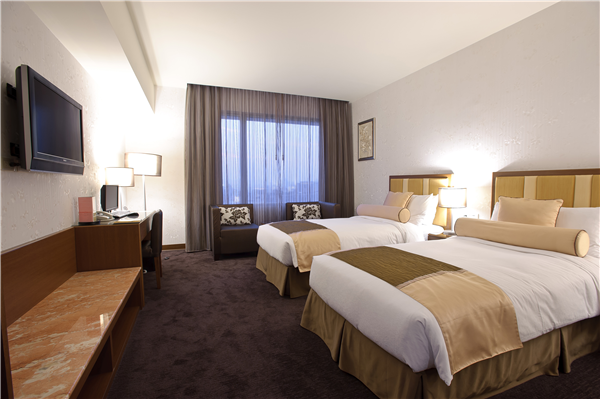客房
•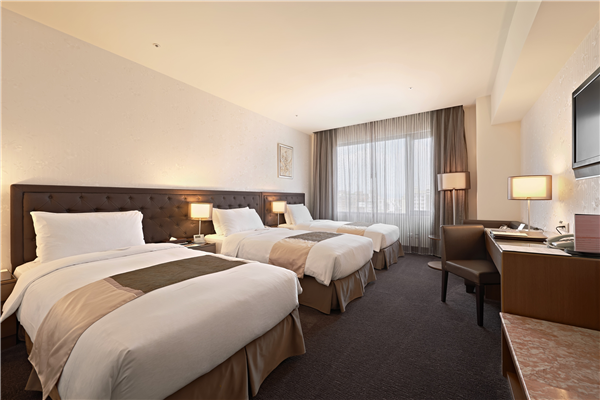客房
•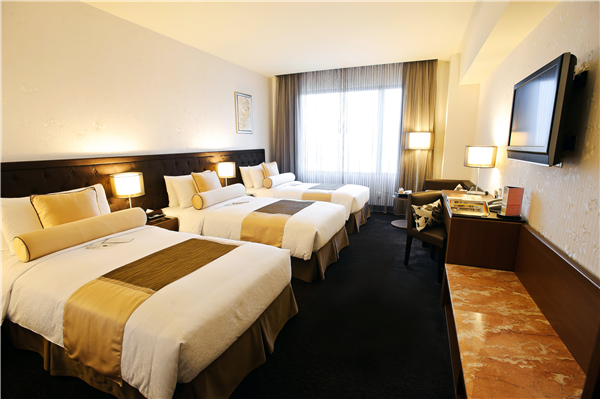客房
•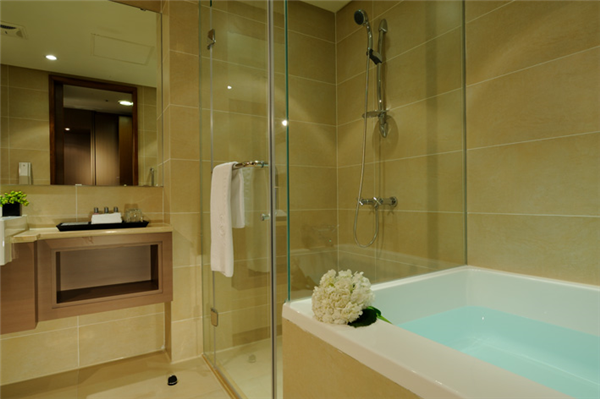客房
•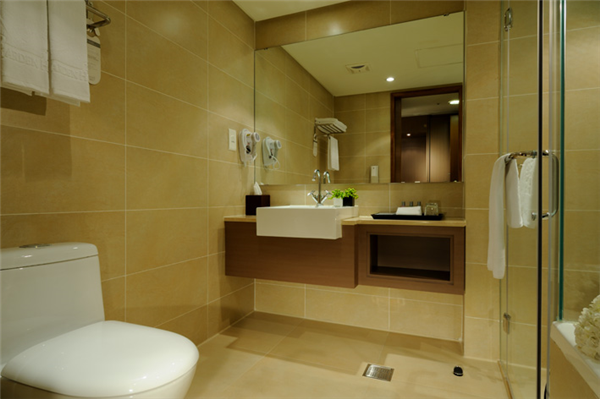客房
•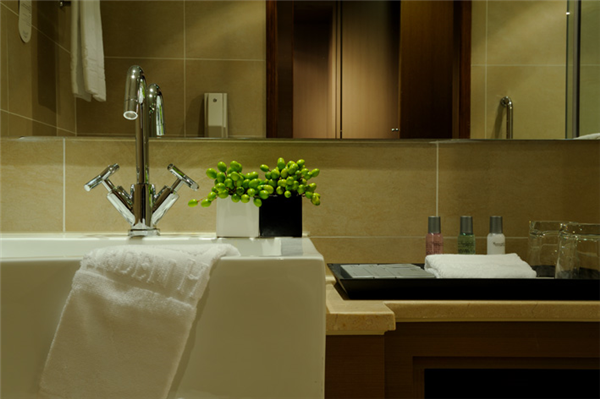客房

飯店設有　 飯店未設 飯店設施

• 24小時客房服務
• 商務中心
• 洗衣服務/乾洗
• Wi-Fi in public areas
• 夜總會
• 禮賓接待服務
• 代客泊車
• 娛樂場
• 自行車出租服務
• 保管服務
• 客房服務
• 行政樓層
• 停車場
• 家庭房
• 酒吧
• 兒童看護服務
• 接送服務
• 電梯
• 可帶寵物
• 旅遊服務
• 飯店/機場接送
• 吸煙房
• 會議設施
• 餐廳
• 咖啡店
• 殘障人士設施
• 髮廊
• 商店
• 池畔吧

• DVD/CD播放器
• 小廚房
• 無線上網(收費)
• 休憩區
• 微波爐
• 燙衣板
• 免費瓶裝水
• 房內保險箱
• 獨立淋浴間
• 公共浴室
• 房內遊戲機
• 獨立用餐區
• 冰箱
• 手提電腦保險箱
• 禁煙房
• 冷氣
• 按摩浴缸
• 私人泳池
• 加長的床(>2m)
• 日報
• 茶與咖啡沖泡設備
• 可提供相連房間
• 書桌
• 衛星頻道/有線電視
• 吹風機
• 洗衣機
• 迷你吧
• 寬頻上網
• 浴室
• 陽台/露台
• 寬頻上網(免費)
• 浴缸
• 電影點播服務
• 寬頻上網(收費)
• 浴袍
• 電視
• 寬頻上網(無線)
• 液晶電視
• 電風扇
• 尊用休息室
• 無線上網(免費)

• spa
• 三溫暖/水療設備
• 網球場
• 健身房/健身設施
• 水上活動
• 花園
• 兒童娛樂室
• 水上運動項目(非機動項目)
• 蒸浴室
• 壁球場
• 溫泉
• 遊戲室/娛樂場
• 室內游泳池
• 游泳池(兒童)
• 高爾夫球場(3km內)
• 室外游泳池
• 漩渦按摩浴池
• 高爾夫球場(飯店)
• 按摩
• 私人海灘

飯店條款

• 加床政策根據您所選定的客房而有所不同，更多詳情請查看您所選定的客房的政策

相關資訊

內設停車場 No Yes 1.0Km 241間 Yes 2000TWD 2008 45.0Km 15 550TWD 0TWD 5 12:00 PM 1 60分鐘 110V 2013

0
0